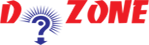﻿ Python Program: How to Add Two Numbers?## Python Program To Add Two Numbers

In this Python Program, you'll learn that How to add two numbers and display it using print() function as well.

Here in this program, you need to use the arithmetic operator (+) to add two numbers.

``````
Python Code
# This program adds two numbers

num1 = 1.5
num2 = 6.3

# Add two numbers
sum = float(num1) + float(num2)

# Display the sum
print('The sum of {0} and {1} is {2}'.format(num1, num2, sum))

Output
The sum of 2.5 and 8.3 is 10.8
```
```

Always remember, to get accurate answer when working with Python, use the float type:

``````
Source Code
# Store input numbers
num1 = input('Enter first number: ')
num2 = input('Enter second number: ')

# Add two numbers
sum = float(num1) + float(num2)

# Display the sum
print('The sum of {0} and {1} is {2}'.format(num1, num2, sum))

Output

Enter first number: 2.5
Enter second number: 8.3
The sum of 2.5 and 8.3 is 10.8
```
```

Here, in this program you need to enter the 2 numbers and this program displays the sum of two numbers that you have entered.

Python built-in function input() takes the input. input() returns it to string and the program converts it into the using using float() function.

Moreover, there is an alternative method too by which you can perform this function in a single statementwithout using any variables.

``````
print('The sum is %.1f' %(float(input('Enter first number: '))+float(input('Enter second number: '))))
```
```

## Other Python Programs

PythonTraining, 258-FF , Katewa Nagar, New Sanganer Rd, Jaipur, 302019